High School+ Needs Assessment
This assessment is based upon Common Core Standards to effectively determine areas of strength and weakness in Secondary Education Mathematics' foundations.
Email *
What is your main goal for tutoring?
What is your parent's phone number and email address? (If under 18) *
What math class will you be / are you taking? *
1 *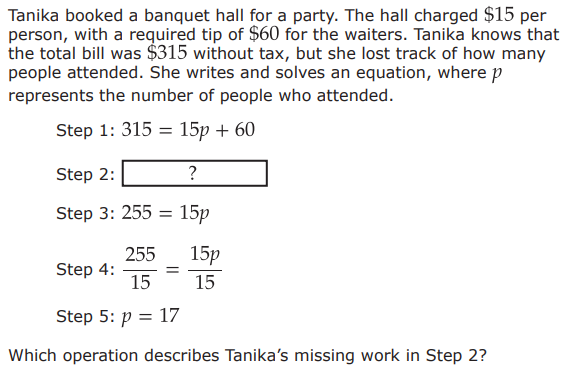2 *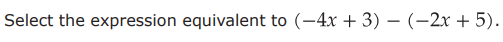3 *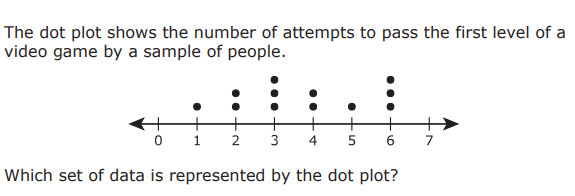4 *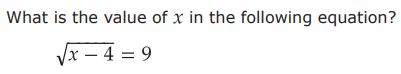5 *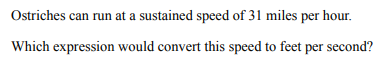6 *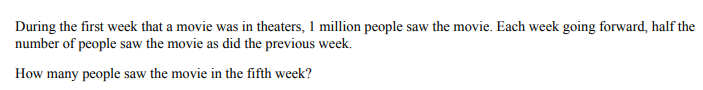7) Simplify the expression *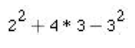8 *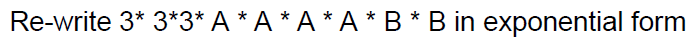9 *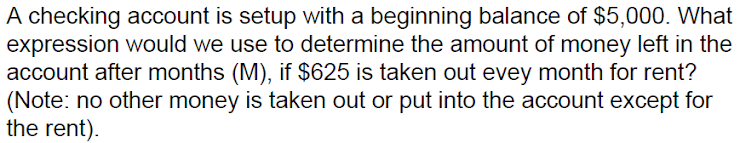10 *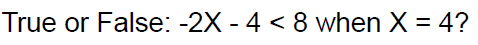11 *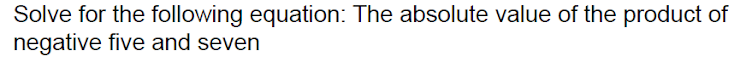12 *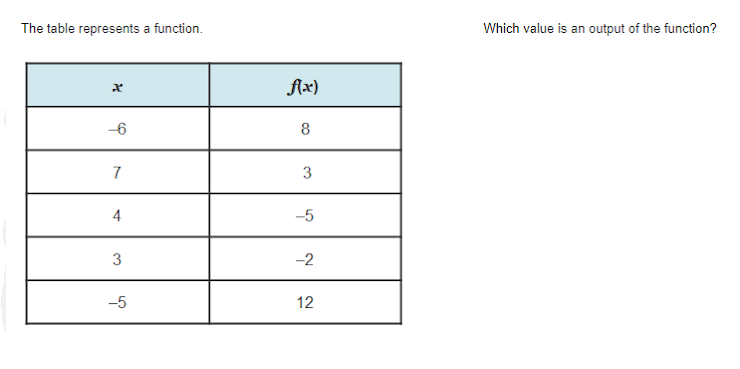13) Which value is an input of the function? *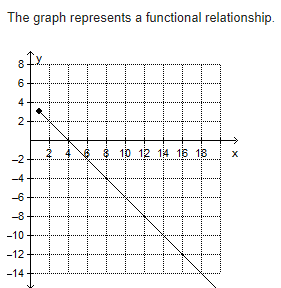14 *15) The solutions to the graphed inequality are shaded on the graph. Which point is a solution? *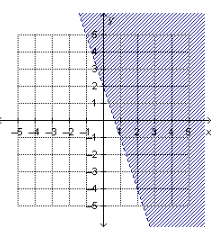16) What is the y-intercept of the function f(x)=4 – 5x? *
17) *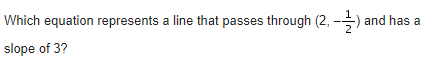18) Which is the solution to the linear equation?            6k + 10.5 = 3k + 12 *
19) What is the solution to the linear equation? *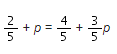20) The volume, V, of a rectangular prism is determined using the formula V=lwh, where l is the length, w is the width, and h is the height of the prism. Using this formula, what is the width of a rectangular prism that has a volume of 138.24 cubic inches, a height of 9.6 inches, and a length of 3.2 inches? Round to the nearest tenth. *
21) The area, a, of an ellipse can be determined using the formula a = πxy, where x and y are half the lengths of the largest and smallest diameters of the ellipse. Which is an equivalent equation solved for y? *
22) Which equation represents the line that passes through (–6, 7) and (–3, 6)? *
23) Which of these values could be the slope of the line? Select two options. *
Required
24) *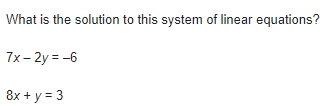Submit
Clear form